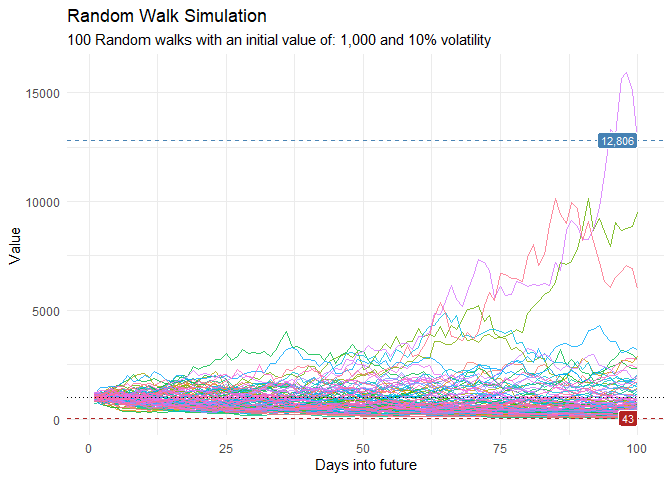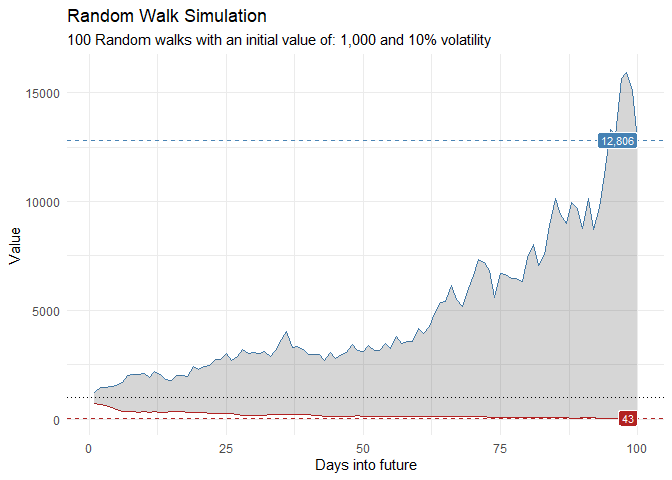The goal of healthyR.ts is to provide a consistent verb framework for performing time series analysis and forecasting on both administrative and clinical hospital data.

## Installation

You can install the released version of healthyR.ts from CRAN with:

install.packages("healthyR.ts")

And the development version from GitHub with:

# install.packages("devtools")
devtools::install_github("spsanderson/healthyR.ts")

## Example

This is a basic example which shows you how to generate random walk data.

library(healthyR.ts)
library(ggplot2)

df <- ts_random_walk()

#> # A tibble: 6 × 4
#>     run     x       y cum_y
#>   <dbl> <dbl>   <dbl> <dbl>
#> 1     1     1  0.175  1175.
#> 2     1     2  0.0481 1231.
#> 3     1     3 -0.195   991.
#> 4     1     4 -0.235   759.
#> 5     1     5 -0.157   640.
#> 6     1     6  0.108   708.

Now that the data has been generated, lets take a look at it.

df %>%
ggplot(
mapping = aes(
x = x
, y = cum_y
, color = factor(run)
, group = factor(run)
)
) +
geom_line(alpha = 0.8) +
ts_random_walk_ggplot_layers(df)That is still pretty noisy, so lets see this in a different way. Lets clear this up a bit to make it easier to see the full range of the possible volatility of the random walks.

library(dplyr)
library(ggplot2)

df %>%
group_by(x) %>%
summarise(
min_y = min(cum_y),
max_y = max(cum_y)
) %>%
ggplot(
aes(x = x)
) +
geom_line(aes(y = max_y), color = "steelblue") +
geom_line(aes(y = min_y), color = "firebrick") +
geom_ribbon(aes(ymin = min_y, ymax = max_y), alpha = 0.2) +
ts_random_walk_ggplot_layers(df)